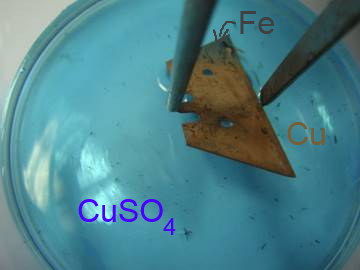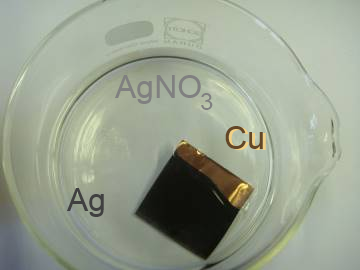# The voltage series

## Definition

The voltage series is the redox table showing the metals(reductants, reducing agents) and their usual cations (oxidants, oxidizing agents) including the couple ($H^+$/$H_2$)

 $Au^{3+}+3e^-$$Au$ $Hg^{2+}+2e^-$$Hg$ $Ag^{+}+e^-$$Ag$ $Cu^{2+}+2e^-$$Cu$ $2H^{+}+2e^-$$H_2$ $Pb^{2+}+2e^-$$Pb$ $Sn^{2+}+2e^-$$Sn$ $Fe^{2+}+2e^-$$Fe$ $Zn^{2+}+2e^-$$Zn$ $Al^{3+}+3e^-$$Al$ $Mg^{2+}+2e^-$$Mg$ $Na^{+}+e^-$$Na$ $Ca^{2+}+2e^-$$Ca$ $K^{+}+e^-$$K$ $Li^{+}+e^-$$Li$

Each oxidant (left) can react with each reductant (right) situated below

## Examples

Iron and copper(II) cation:An iron rod introduced in a solution of copper(II) sulfate becomes covered with a red copper layer:
$Cu^{2+}+2e^- \longrightarrow Cu$
$Fe-2e^- \longrightarrow Fe^{2+}$
______________________________
$Cu^{2+}+Fe \longrightarrow Cu + Fe^{2+}$

Copper and silver cation:A copper sheet introduced in a silver nitrate solution becomes covered with a black silver layer:
$Ag^++e^-\longrightarrow Ag$
$Cu-2e^-\longrightarrow Cu^{2+}$
______________________________
$Cu +2Ag^+\longrightarrow Cu^{2+}+2Ag$$Pb^{2+}+2e^-\longrightarrow Pb$
$Zn-2e^-\longrightarrow Zn^{2+}$
$Zn +Pb^{2+}\longrightarrow Zn^{2+}+Pb$